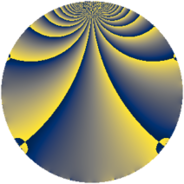Properties

 Label 845.2.mLevel $845$ Weight $2$ Character orbit 845.m Rep. character $\chi_{845}(316,\cdot)$ Character field $\Q(\zeta_{6})$ Dimension $104$ Newform subspaces $10$ Sturm bound $182$ Trace bound $4$

Related objects

Defining parameters

 Level: $$N$$ $$=$$ $$845 = 5 \cdot 13^{2}$$ Weight: $$k$$ $$=$$ $$2$$ Character orbit: $$[\chi]$$ $$=$$ 845.m (of order $$6$$ and degree $$2$$) Character conductor: $$\operatorname{cond}(\chi)$$ $$=$$ $$13$$ Character field: $$\Q(\zeta_{6})$$ Newform subspaces: $$10$$ Sturm bound: $$182$$ Trace bound: $$4$$ Distinguishing $$T_p$$: $$2$$

Dimensions

The following table gives the dimensions of various subspaces of $$M_{2}(845, [\chi])$$.

Total New Old
Modular forms 212 104 108
Cusp forms 156 104 52
Eisenstein series 56 0 56

Trace form

 $$104 q - 2 q^{3} + 54 q^{4} + 18 q^{6} + 6 q^{7} - 52 q^{9} + O(q^{10})$$ $$104 q - 2 q^{3} + 54 q^{4} + 18 q^{6} + 6 q^{7} - 52 q^{9} + 2 q^{10} - 20 q^{12} - 12 q^{14} + 6 q^{15} - 54 q^{16} - 2 q^{17} - 12 q^{19} - 12 q^{20} + 16 q^{22} + 10 q^{23} + 12 q^{24} - 104 q^{25} + 4 q^{27} + 18 q^{28} + 12 q^{29} - 4 q^{30} - 6 q^{32} - 42 q^{33} - 10 q^{35} + 16 q^{36} - 6 q^{37} + 64 q^{38} + 12 q^{40} - 12 q^{41} + 16 q^{42} + 2 q^{43} + 42 q^{46} - 8 q^{48} + 36 q^{49} + 16 q^{51} - 18 q^{54} + 4 q^{55} - 44 q^{56} - 36 q^{58} + 12 q^{59} + 44 q^{61} - 20 q^{62} + 24 q^{63} - 80 q^{64} - 68 q^{66} - 6 q^{67} + 38 q^{68} + 4 q^{69} + 48 q^{72} + 10 q^{74} + 2 q^{75} - 54 q^{76} + 68 q^{77} - 8 q^{79} - 56 q^{81} - 24 q^{82} + 30 q^{84} - 18 q^{85} - 14 q^{87} + 6 q^{88} - 24 q^{89} - 24 q^{90} - 108 q^{92} - 20 q^{94} + 16 q^{95} + 30 q^{97} - 72 q^{98} + O(q^{100})$$

Decomposition of $$S_{2}^{\mathrm{new}}(845, [\chi])$$ into newform subspaces

Label Dim. $$A$$ Field CM Traces $q$-expansion
$a_{2}$ $a_{3}$ $a_{5}$ $a_{7}$
845.2.m.a $4$ $6.747$ $$\Q(\zeta_{12})$$ None $$-6$$ $$-2$$ $$0$$ $$0$$ $$q+(-1-\zeta_{12}^{2})q^{2}+(-1-\zeta_{12}+\zeta_{12}^{2}+\cdots)q^{3}+\cdots$$
845.2.m.b $4$ $6.747$ $$\Q(\zeta_{12})$$ None $$0$$ $$4$$ $$0$$ $$0$$ $$q+\zeta_{12}q^{2}+(2-2\zeta_{12}^{2})q^{3}-\zeta_{12}^{2}q^{4}+\cdots$$
845.2.m.c $4$ $6.747$ $$\Q(\zeta_{12})$$ None $$6$$ $$-2$$ $$0$$ $$0$$ $$q+(2-\zeta_{12}^{2})q^{2}+(\zeta_{12}-\zeta_{12}^{2}+\zeta_{12}^{3})q^{3}+\cdots$$
845.2.m.d $8$ $6.747$ 8.0.592240896.1 None $$0$$ $$-4$$ $$0$$ $$0$$ $$q+\beta _{1}q^{2}-\beta _{3}q^{3}+(1-2\beta _{3}+\beta _{4}+\beta _{6}+\cdots)q^{4}+\cdots$$
845.2.m.e $8$ $6.747$ 8.0.12960000.1 None $$0$$ $$0$$ $$0$$ $$0$$ $$q+\beta _{6}q^{2}+(-1+2\beta _{4}+\beta _{5})q^{3}+(-\beta _{2}+\cdots)q^{4}+\cdots$$
845.2.m.f $8$ $6.747$ $$\Q(\zeta_{24})$$ None $$0$$ $$0$$ $$0$$ $$0$$ $$q+(-\zeta_{24}+\zeta_{24}^{3}+\zeta_{24}^{5})q^{2}-\zeta_{24}^{4}q^{3}+\cdots$$
845.2.m.g $8$ $6.747$ 8.0.22581504.2 None $$0$$ $$2$$ $$0$$ $$6$$ $$q+(\beta _{3}+\beta _{5}+\beta _{7})q^{2}+(1-\beta _{1}-2\beta _{2}+\cdots)q^{3}+\cdots$$
845.2.m.h $12$ $6.747$ $$\mathbb{Q}[x]/(x^{12} - \cdots)$$ None $$0$$ $$4$$ $$0$$ $$0$$ $$q-\beta _{8}q^{2}+(1+\beta _{7}-\beta _{11})q^{3}+(-\beta _{3}+\cdots)q^{4}+\cdots$$
845.2.m.i $12$ $6.747$ 12.0.$$\cdots$$.1 None $$0$$ $$10$$ $$0$$ $$0$$ $$q+\beta _{1}q^{2}+(2-2\beta _{7}+\beta _{9})q^{3}+(1-\beta _{3}+\cdots)q^{4}+\cdots$$
845.2.m.j $36$ $6.747$ None $$0$$ $$-14$$ $$0$$ $$0$$

Decomposition of $$S_{2}^{\mathrm{old}}(845, [\chi])$$ into lower level spaces

$$S_{2}^{\mathrm{old}}(845, [\chi]) \cong$$ $$S_{2}^{\mathrm{new}}(13, [\chi])$$$$^{\oplus 4}$$$$\oplus$$$$S_{2}^{\mathrm{new}}(65, [\chi])$$$$^{\oplus 2}$$$$\oplus$$$$S_{2}^{\mathrm{new}}(169, [\chi])$$$$^{\oplus 2}$$